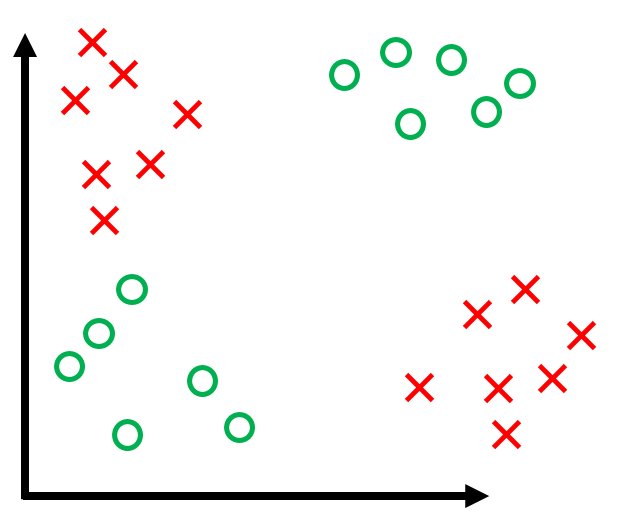## Lecture 3: The Perceptron

Video II

### Assumptions

1. Binary classification (i.e. $y_i \in \{-1, +1\}$)
2. Data is linearly separable

### Classifier

$$h(x_i) = \textrm{sign}(\mathbf{w}^\top \mathbf{x}_i + b)$$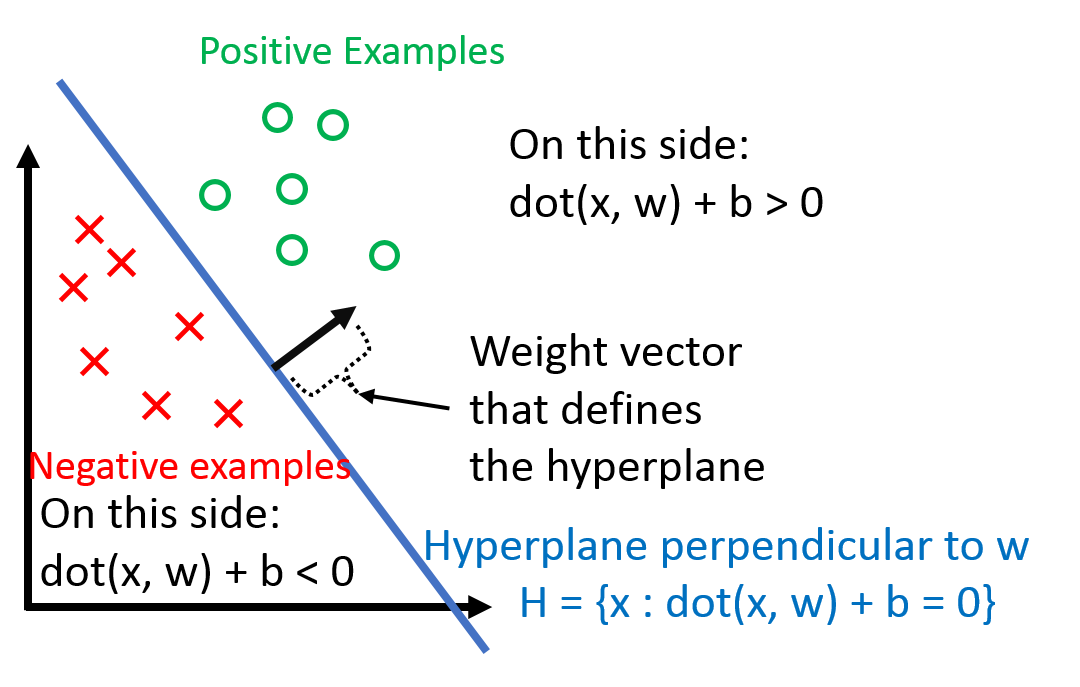$b$ is the bias term (without the bias term, the hyperplane that $\mathbf{w}$ defines would always have to go through the origin). Dealing with $b$ can be a pain, so we 'absorb' it into the feature vector $\mathbf{w}$ by adding one additional constant dimension. Under this convention, $$\mathbf{x}_i \hspace{0.1in} \text{becomes} \hspace{0.1in} \begin{bmatrix} \mathbf{x}_i \\ 1 \end{bmatrix} \\ \mathbf{w} \hspace{0.1in} \text{becomes} \hspace{0.1in} \begin{bmatrix} \mathbf{w} \\ b \end{bmatrix} \\$$ We can verify that $$\begin{bmatrix} \mathbf{x}_i \\ 1 \end{bmatrix}^\top \begin{bmatrix} \mathbf{w} \\ b \end{bmatrix} = \mathbf{w}^\top \mathbf{x}_i + b$$ Using this, we can simplify the above formulation of $h(\mathbf{x}_i)$ to $$h(\mathbf{x}_i) = \textrm{sign}(\mathbf{w}^\top \mathbf{x})$$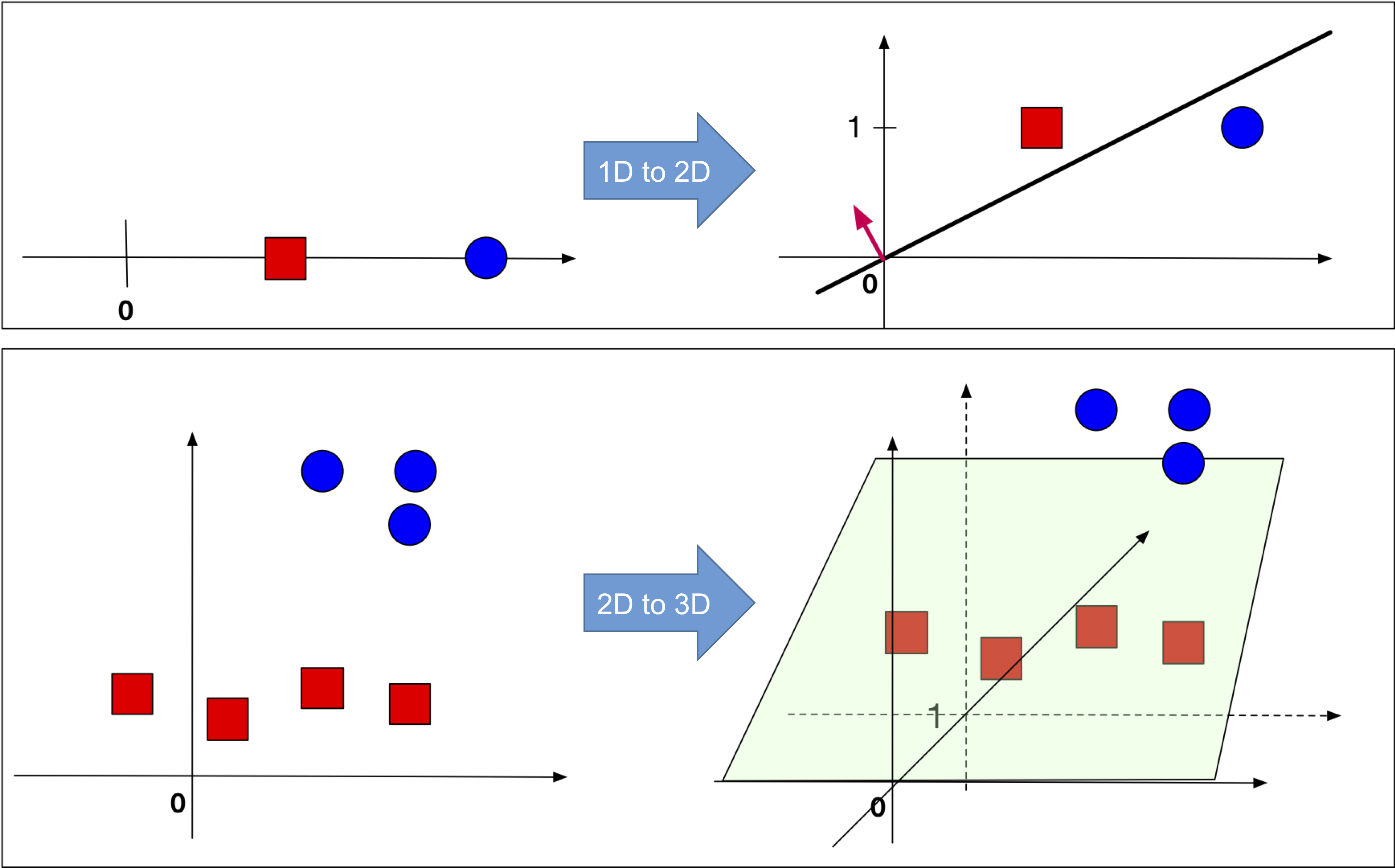(Left:) The original data is 1-dimensional (top row) or 2-dimensional (bottom row). There is no hyper-plane that passes through the origin and separates the red and blue points. (Right:) After a constant dimension was added to all data points such a hyperplane exists.
Observation: Note that $$y_i(\mathbf{w}^\top \mathbf{x}_i) > 0 \Longleftrightarrow \mathbf{x}_i \hspace{0.1in} \text{is classified correctly}$$ where 'classified correctly' means that $x_i$ is on the correct side of the hyperplane defined by $\mathbf{w}$. Also, note that the left side depends on $y_i \in \{-1, +1\}$ (it wouldn't work if, for example $y_i \in \{0, +1\}$).

### Perceptron Algorithm

Now that we know what the $\mathbf{w}$ is supposed to do (defining a hyperplane the separates the data), let's look at how we can get such $\mathbf{w}$.

Perceptron Algorithm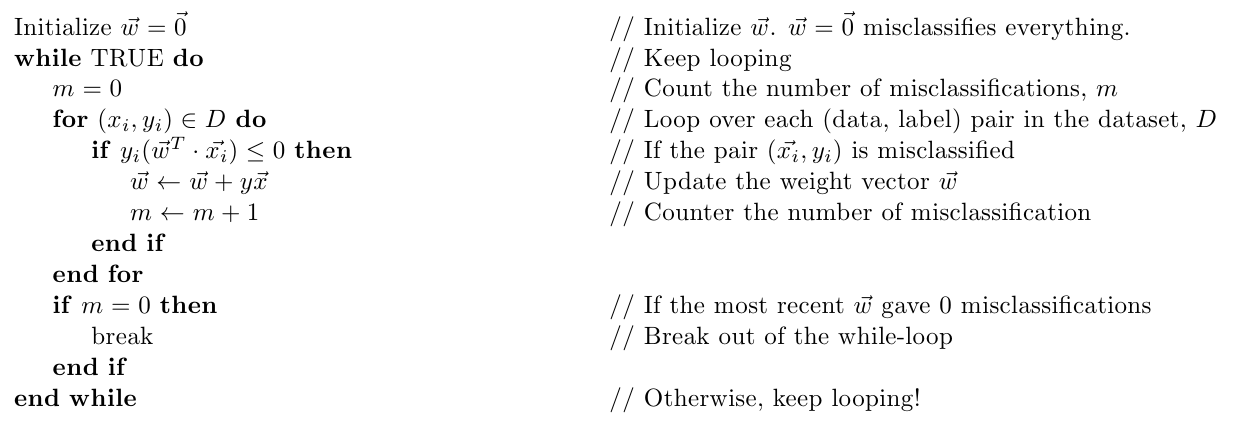#### Geometric Intuition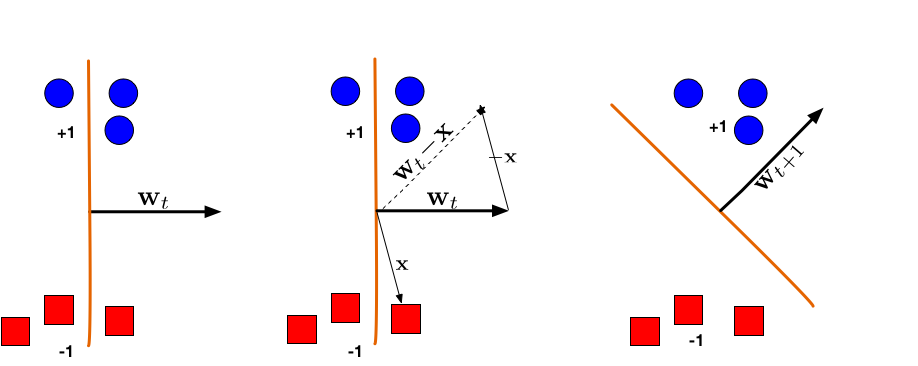Illustration of a Perceptron update. (Left:) The hyperplane defined by $\mathbf{w}_t$ misclassifies one red (-1) and one blue (+1) point. (Middle:) The red point $\mathbf{x}$ is chosen and used for an update. Because its label is -1 we need to subtract $\mathbf{x}$ from $\mathbf{w}_t$. (Right:) The udpated hyperplane $\mathbf{w}_{t+1}=\mathbf{w}_t-\mathbf{x}$ separates the two classes and the Perceptron algorithm has converged.

Quiz: Assume a data set consists only of a single data point $\{(\mathbf{x},+1)\}$. How often can a Perceptron misclassify this point $\mathbf{x}$ repeatedly? What if the initial weight vector $\mathbf{w}$ was initialized randomly and not as the all-zero vector?

### Perceptron Convergence

The Perceptron was arguably the first algorithm with a strong formal guarantee. If a data set is linearly separable, the Perceptron will find a separating hyperplane in a finite number of updates. (If the data is not linearly separable, it will loop forever.)

The argument goes as follows: Suppose $\exists \mathbf{w}^*$ such that $y_i(\mathbf{x}^\top \mathbf{w}^* ) > 0$ $\forall (\mathbf{x}_i, y_i) \in D$.

Now, suppose that we rescale each data point and the $\mathbf{w}^*$ such that $$||\mathbf{w}^*|| = 1 \hspace{0.3in} \text{and} \hspace{0.3in} ||\mathbf{x}_i|| \le 1 \hspace{0.1in} \forall \mathbf{x}_i \in D$$ Let us define the Margin $\gamma$ of the hyperplane $\mathbf{w}^*$ as $\gamma = \min_{(\mathbf{x}_i, y_i) \in D}|\mathbf{x}_i^\top \mathbf{w}^* |$.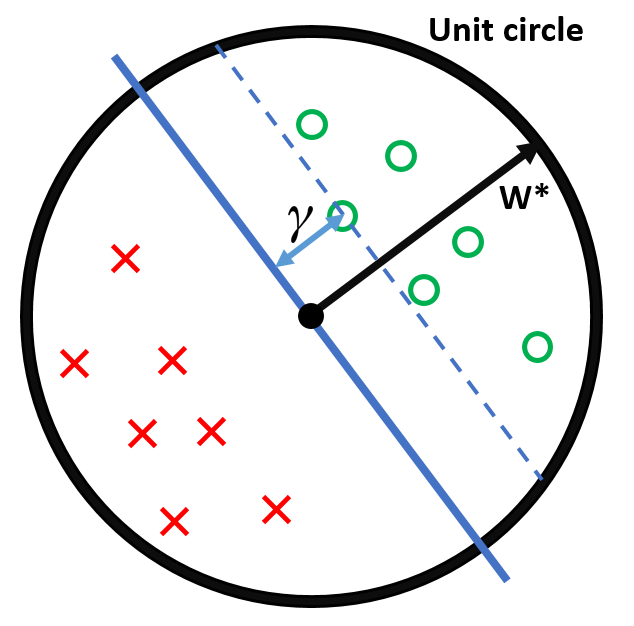To summarize our setup:
• All inputs $\mathbf{x}_i$ live within the unit sphere
• There exists a separating hyperplane defined by $\mathbf{w}^*$, with $\|\mathbf{w}\|^*=1$ (i.e. $\mathbf{w}^*$ lies exactly on the unit sphere).
• $\gamma$ is the distance from this hyperplane (blue) to the closest data point.

Theorem: If all of the above holds, then the Perceptron algorithm makes at most $1 / \gamma^2$ mistakes.

Proof:
Keeping what we defined above, consider the effect of an update ($\mathbf{w}$ becomes $\mathbf{w}+y\mathbf{x}$) on the two terms $\mathbf{w}^\top \mathbf{w}^*$ and $\mathbf{w}^\top \mathbf{w}$. We will use two facts:
• $y( \mathbf{x}^\top \mathbf{w})\leq 0$: This holds because $\mathbf x$ is misclassified by $\mathbf{w}$ - otherwise we wouldn't make the update.
• $y( \mathbf{x}^\top \mathbf{w}^*)>0$: This holds because $\mathbf{w}^*$ is a separating hyper-plane and classifies all points correctly.
1. Consider the effect of an update on $\mathbf{w}^\top \mathbf{w}^*$: $$(\mathbf{w} + y\mathbf{x})^\top \mathbf{w}^* = \mathbf{w}^\top \mathbf{w}^* + y(\mathbf{x}^\top \mathbf{w}^*) \ge \mathbf{w}^\top \mathbf{w}^* + \gamma$$ The inequality follows from the fact that, for $\mathbf{w}^*$, the distance from the hyperplane defined by $\mathbf{w}^*$ to $\mathbf{x}$ must be at least $\gamma$ (i.e. $y (\mathbf{x}^\top \mathbf{w}^*)=|\mathbf{x}^\top \mathbf{w}^*|\geq \gamma$).

This means that for each update, $\mathbf{w}^\top \mathbf{w}^*$ grows by at least $\gamma$.
2. Consider the effect of an update on $\mathbf{w}^\top \mathbf{w}$: $$(\mathbf{w} + y\mathbf{x})^\top (\mathbf{w} + y\mathbf{x}) = \mathbf{w}^\top \mathbf{w} + \underbrace{2y(\mathbf{w}^\top\mathbf{x})}_{<0} + \underbrace{y^2(\mathbf{x}^\top \mathbf{x})}_{0\leq \ \ \leq 1} \le \mathbf{w}^\top \mathbf{w} + 1$$ The inequality follows from the fact that
• $2y(\mathbf{w}^\top \mathbf{x}) < 0$ as we had to make an update, meaning $\mathbf{x}$ was misclassified
• $0\leq y^2(\mathbf{x}^\top \mathbf{x}) \le 1$ as $y^2 = 1$ and all $\mathbf{x}^\top \mathbf{x}\leq 1$ (because $\|\mathbf x\|\leq 1$).
3. This means that for each update, $\mathbf{w}^\top \mathbf{w}$ grows by at most 1.
4. Now we know that after $M$ updates the following two inequalities must hold:
• (1) $\mathbf{w}^\top\mathbf{w}^*\geq M\gamma$
• (2) $\mathbf{w}^\top \mathbf{w}\leq M$.
We can then complete the proof: \begin{align} M\gamma &\le \mathbf{w}^\top \mathbf{w}^* &&\text{By (1)} \\ &=\|\mathbf{w}\|\cos(\theta) && \text{by definition of inner-product, where $\theta$ is the angle between $\mathbf{w}$ and $\mathbf{w}^*$.}\\ &\leq ||\mathbf{w}|| &&\text{by definition of $\cos$, we must have $\cos(\theta)\leq 1$.} \\ &= \sqrt{\mathbf{w}^\top \mathbf{w}} && \text{by definition of $\|\mathbf{w}\|$} \\ &\le \sqrt{M} &&\text{By (2)} \\ & \textrm{ }\\ &\Rightarrow M\gamma \le \sqrt{M} \\ &\Rightarrow M^2\gamma^2 \le M \\ &\Rightarrow M \le \frac{1}{\gamma^2} && \text{And hence, the number of updates $M$ is bounded from above by a constant.} \end{align}

Quiz: Given the theorem above, what can you say about the margin of a classifier (what is more desirable, a large margin or a small margin?) Can you characterize data sets for which the Perceptron algorithm will converge quickly? Draw an example.

### History

• Initially, huge wave of excitement ("Digital brains") (See The New Yorker December 1958)
• Then, contributed to the A.I. Winter. Famous example of a simple non-linearly separable data set, the XOR problem (Minsky 1969):
•